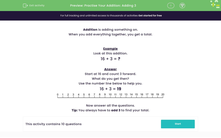### Comprehensive & curriculum aligned

In this worksheet, students practise adding 3 to numbers in the range 0-20.Key stage:  KS 1

Curriculum topic:   Number: Addition and Subtraction

Curriculum subtopic:   Add and Subtract to 20

Difficulty level:#### Worksheet Overview

When you add everything together, you get a total.

Example

16 + 3 = ?

Start at 16 and count 3 forward.

What do you get then?

16 + 3 = 19